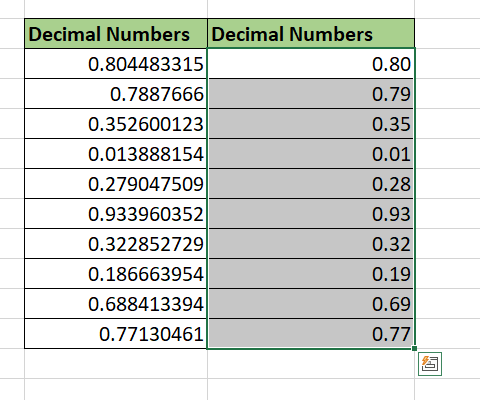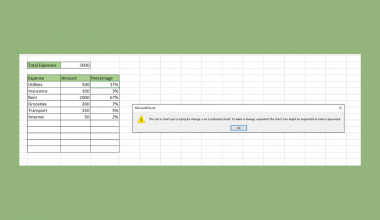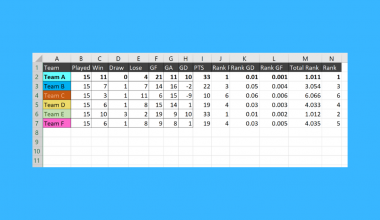# How to Round Numbers Without Formula in Excel

This guide will explain how to round numbers without formulas in Excel.

Excel provides several formatting options that will display decimal values with a specified number of decimal places.

When working with data, you may want to round decimal values for various reasons.

For example, most financial transactions only need values with up to two decimal places. Spreadsheets may also be more difficult to understand or navigate if values have more than three decimal places.

Excel includes a few functions that can round numerical data. These formulas include `TRUNC`, `ROUNDUP`, `ROUNDDOWN`, and `MROUND`

However, Excel also provides options to round numbers that do not require formulas.

With the built-in cell formatting features, the user can format a cell or cell range to display only a limited number of decimal places.

Note that rounding numbers through formatting only changes how the value is displayed. Formatting does not modify the value itself.

Now that we have a good idea of when to round numbers without using formulas, let’s learn how to use it and work on an actual sample spreadsheet.

## A Real Example of Rounding Numbers Without Using a Formula

The following section provides several examples of how to round numbers without using a formula. We will also go into detail about the formulas and tools used in these examples.

First, let’s take a look at a real example of how we can round a range of decimal values without using a formula.

The sample data below shows ten decimal values with seven or more decimal places. We want to round these values to the nearest hundredths place. This should leave us with two digits after the decimal point.We can use Excel’s built-in cell formatting features to change how the spreadsheet displays the number.Do you want to take a closer look at our examples? You can make your own copy of the spreadsheet above using the link attached below.

Use our sample spreadsheet to see how the cell formatting does not actually change the numerical value.

If you’re ready to try out rounding numbers without formulas in Excel, head over to the next section to read our step-by-step breakdown on how to do it!

## How to Round Numbers Without Using a Formula in Excel

This section will guide you through each step needed to round numbers in Excel without using a formula. You’ll learn how we can use the Decrease Decimal shortcut and custom number formatting to round decimals to the desired significance.

Follow these steps to start rounding numbers in Excel without using formulas:

1. First, select the cell or cell range that you want to round up. In this example, we’ll round numbers in the range C3:C12.2. Next, head to the Home tab and click on the Decrease Decimal option in the Number group.3. Your cell or cell range should now be rounded up. You may continue to click on the shortcut until you reach your desired number of decimal places. In this example, we’ve decreased the number of decimal places to two.4. Next, we’ll try to use cell formatting to round our selected range to a specified number of decimal places. Select the cell or cell range you want to format and right-click on the selection. Click on the option Format Cells.5. In the Format Cells dialog box, navigate to the Number tab. Select the Number category from the list of categories. Select the number of decimal places using the number picker seen below. Click on OK to apply this formatting.6. The selected cell range should now be rounded up. In this example, we’ve decreased the number of decimal places to two.7. Next, we’ll show you how to use custom cell formatting to indicate the number of decimal places to display. Select the cell or cell range you want to format and right-click on the selection. Click on the Format Cells option to access the cell formatting settings.8. In the Number tab, click on the Custom category. In the text box under Type, the user must indicate the number of decimal places to show by typing the desired number format code. For example, if the user wants to show four decimal places, they may use the format code ‘0.0000’These are all the steps needed to round numbers in Excel without using a formula.

This step-by-step guide is a quick introduction to using cell formatting features to round numbers without a formula in Excel.

You should now understand how to access the Format Cell dialog box and use a preset option or create your own custom number format code.

This function is just one example of the many Excel functions you can use in your spreadsheets. Our website offers hundreds of other functions and methods to help you get more out of Microsoft Excel.

With so many other Excel functions available, you can find one appropriate for your use case.

Don’t miss out on our team’s new spreadsheet tips, tricks, and best practices. Subscribe to our newsletter to stay updated on the latest guides from us!### Get emails from us about Excel.

Our goal this year is to create lots of rich, bite-sized tutorials for Excel users like you. If you liked this one, you'd love what we are working on! Readers receive ✨ early access ✨ to new content.

##### You May Also Like## How to Unlock Grayed Out Menus in Excel

This guide will explain how you can try multiple methods to unlock grayed out menus in Excel. Some…## How to Automatically Fill Increment Cells in Excel

This guide will show you how you can automatically fill increment cells in Excel. We commonly increment cells…## How to Use a Data Model in Excel

This guide will explain how to start using a data model in Excel. Excel’s Data Model tool is…## How to Protect Formulas Without Protecting Worksheet in Excel

This guide will explain how you can protect formulas without protecting worksheets in Excel. You can protect cells…